# AP Calculus AB : How to find maximum values

## Example Questions

### Example Question #1 : How To Find Maximum Values

What is the local maximum of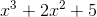when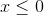?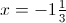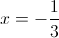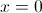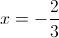There is no local maximum.Explanation:

To find the maximum, we need to look at the first derivative.

To find the first derivative, we can use the power rule. To do that, we lower the exponent on the variables by one and multiply by the original exponent.

We're going to treatas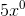since anything to the zero power is one.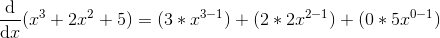Notice that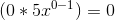since anything times zero is zero.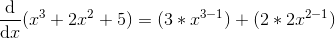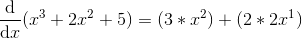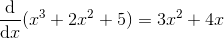When looking at the first derivative, remember that if the output of this equation is positive, the original function is increasing. If the derivative is negative, then the function is decreasing.

Notice that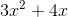changes from positive to negative when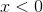.

We can find that root using the quadratic equation: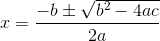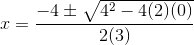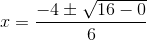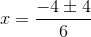Since we're looking for a negative value, we will subtract.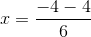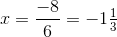Therefore, the maximum is at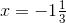.

### Example Question #2 : How To Find Maximum Values

What is the local maximum of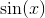between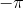and?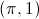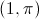There is no maximum between these two points.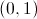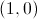Explanation:

To find the maximum, we must find where the graph shifts from increasing to decreasing. To find out the rate at which the graph shifts from increasing to decreasing, we look at the second derivative and see when the value changes from positive to negative.

That is to say, we will look at the second derivative and see where (if at all) the graph crosses the x-axis and is moving from a positive y-value to a negative y-value.

Now we must find the second derivative. Unfortunately, the derivatives of trig functions must be memorized. The first derivative is: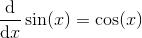.

To find the second derivative, we take the derivative of our result.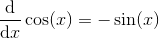.

Therefore, the second derivative will be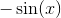.

Does our new equation cross the x-axis and move from positive to negative betweenand? Yes. It does once, when. Therefore, our local maximum shall be when. Plug that value back into our first equation to find that the maximum will be at the point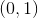.

### Example Question #3 : How To Find Maximum Values

Find the x coordinate of the maximum of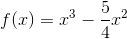over the interval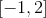.

6

-2

2

5

0

2

Explanation:

First find the derivative, which is: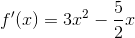Set that equal to zero to get your critical points: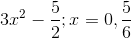You also have to take into account -1 and 2 as critical points since those are your endpoints. Evaluate each of these critical points in the original function: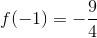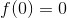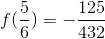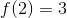The greatest is your maximum. The x coordinate is 2.

### Example Question #4 : How To Find Maximum Values

Find the value at which the function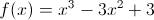reaches its local maximum.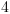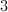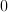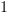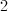Explanation:

To find the maximum, we need to find the critical points. To do that, we need to take the derivatie of the function.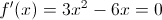And we can see that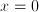and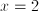are critical points for this function.

An easy way to see which is the maximum and which is the minimum is to plug in the values of the critical points into the original equation.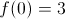and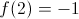Therefore, the maximum value is 3, and the value at which the function reaches the maximum is 0.